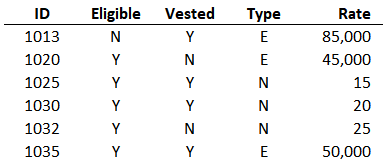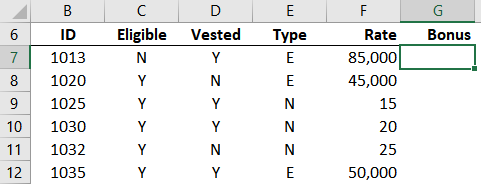## SWITCH or IFS

In the previous post, we learned that the SWITCH function allows us to look at a value and then return different results based on that value. But, what if instead of looking at one specific value, we need to consider multiple values in order to determine which result to return?

Traditionally, we may have approached this by nesting multiple IF functions together. But, we now have an additional option: the IFS function.

So, from a high level, we can use SWITCH when we need to examine a single value (cell, expression), and IFS when we need to examine multiple values (cells, expressions). Let’s dig in.

This post is brought to you by Dropbox. Before we get to the mechanics of the IFS function, I’d like to personally thank Dropbox for sponsoring this post. There is one specific Dropbox feature I’d like to highlight because it can be a lifesaver. It is the ability to recover a previous version of a file. So, say we are working on an Excel workbook. We make some changes and then Save and Close the workbook. Later, we panic when we realize we need the prior version of the workbook. If the file was stored with Dropbox, recovering the prior version would be no problem. If you’d like to learn more, check out the file recovery feature here:

## Overview

Let’s say we need to compute a bonus of 5% of the annual pay for certain employees. Here is our list of employees:To compute the bonus, we need to first consider if the employee is Eligible. If they are not Eligible (N), then this bonus does not apply and the formula should return a \$0 bonus amount. If they are Eligible, then we need to determine if they are Vested in the bonus program. If they are not Vested (N), then the formula should return \$0 bonus. If they are Vested, then we need to compute the bonus amount at 5% of their annual pay. If Type is E, then the employee is Exempt and their Rate is expressed as an annual salary. If Type is N, then they are Nonexempt and the Rate is expressed as an hourly rate which needs to be annualized to compute the bonus amount.

Historically, we could approach this by nesting multiple IF functions inside each other, something like this =IF(Eligible=”N”, 0, IF(Vested=”N”, 0, IF(Type=”E”, …  and so on. But, starting with Excel for Windows O365 / Excel 2019, we have another option: IFS.

## IFS

The IFS function is like the plural of the IF function and supports multiple tests. So, depending on your workbook, IFS may be an nice alternative to using many nested IF functions.

Essentially, we list pairs of test/result arguments in the IFS function. The result for the first test in the list that is found to be TRUE is returned. The final pair can be used to return a value when none of the other tests are TRUE (think of it like setting the value to return if all other tests are FALSE).

So, the IFS function looks a bit like this:

`=IFS(test1,value_if_true1, test2,value_if_true2, ..., TRUE, result_if_others_are_false)`

Now that we have a basic understanding of how the function works, let’s apply it to our bonus calculation.

Our goal is to write a formula in G7 that we can fill down to compute the Bonus for each employee. Here is our list of employees:We’ll build the formula one step at a time. First, we need to determine if the employee is Eligible. If so, we’d like to continue on to the next test, but if not, then we want to stop immediately and return 0. So, we basically start with the following:

`=IFS(C7="N",0,`

We have our first pair of arguments. The first argument is the test and the second is what to return if the test is TRUE. So, if the employee is not Eligible (C7=”N”), then we want to stop testing and return 0. Otherwise, it will continue to the next test.

The next test needs to determine if the employee is Vested in this bonus program. If so, continue to the next test otherwise stop and return 0. So, we add another pair of arguments to our formula like this:

`=IFS(C7="N",0, D7="N",0,`

If we pass this test, it means the employee gets a bonus of 5% of their annual pay. But, computing that requires yet another test because the annual pay calculation depends on the Type. If the Type is E (Exempt) then the bonus amount is 5% of the Rate (because the rate is the annual salary). So, we update our formula with an additional pair of arguments as follows:

`=IFS(C7="N",0, D7="N",0, E7="E",F7*.05,`

The final calculation needs to address Nonexempt employees (Type is N). When Type is N, we need to multiply the hourly rate by 2080 hours to determine the annual pay. We have a couple of options here.

One option would be to add a specific test like E7=”N” … and that would be just fine. But, I’d like to demonstrate another option which can be used to return a value if all previous tests are false. To do this, we just enter a test value that will return TRUE, and then the result. So, something like this would work:

`=IFS(C7="N",0, D7="N",0, E7="E",F7*.05, TRUE, F7*2080*.05)`

We fill the formula down and bam:Since this is Excel, there are multiple ways to accomplish just about anything … including this bonus calculation. With the introduction of IFS, we have an option that can simplify formulas that have historically required several nested IF functions.

If you have any other IFS tips, please share by posting a comment below … thanks!

Sample file: IFS.xlsx

## Disclosures and Notes

• This is a sponsored post for Dropbox. All opinions are my own. Dropbox is not affiliated with nor endorses any other products or services mentioned.
• 100% of sponsor proceeds fund the Excel University scholarship and financial aid programs … thank you!

Posted in ,### Jeff Lenning

I love sharing the things I've learned about Excel, and I built Excel University to help me do that. My motto is: Learn Excel. Work Faster.

### Want to learn Excel?

Our Campus Pass includes access to our entire Undergrad and Masters catalog. Gamification ensures it is the most fun you can have learning Excel :)

1.Travis Wallace on June 28, 2019 at 9:35 am

Jeff,

First off, thanks for all the great Excel content! It is proving to be very helpful!

I am confused about the “True” argument you used in the formula (Logical Test 4). What does that do that just coding in a reference to the type cell being equal to “N” and then having the formula calculate the annualized pay amount and bonus amount doesn’t do? Is it just a way to keep the formula moving to the logical conclusion without it having to actually evaluate anything?

Thanks again!

•Jeff Lenning on July 1, 2019 at 8:13 am

By using True (or any value or expression that returns True) we tell the function to return that final value if no other arguments are true. It is basically setting up the “else” logic, which says if nothing else is true then return this final value.
Thanks
Jeff

•Travis Wallace on July 2, 2019 at 6:31 pm

Thanks for responding, Jeff. I understand now!

Thanks!

!
!